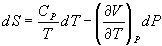5HC - October 1999

Part A (20 x 2 = 40 Marks)

1. What is path function?
2. Explain internal energy
3. When will be the kinetic energy of molecules zero?
4. What are the various steps involved in a Carnot cycle?
5. Define entropy
6. What is compressibility factor?
7. Explain reversible process
8. What is an open system?
9. Define third law of thermodynamics
10. Explain the multistage compression
11. Define excess property
12. Explain C.O.P
13. Define one ton of refrigeration
14. Define standard heat of reaction
15. Explain equilibrium constant
16. What is heat pump?
17. Explain activity
18. State Vander Waals equation of state
19. Define chemical potential
20. What is the significance of van Laar equation?
21. Part B (5 x 12 = 60 Marks)

22. (a) Derive the first law of thermodynamics for open system (5)
23. (b) Gas is compressed at the rate of 0.2 m3/min (25oC, 1 atm) to 50 atm. If heat losses during the operation are 1 kcal/min, what is the enthalpy change? State assumptions (7)

Or

24. (a) Define absolute temperature (3)
25. (b) A reversible heat engine absorbs 1000 kcal of heat at 327oC and produces work and discards heat at 29oC. What is the change in entropy of heat source and heat sink? What is the total entropy change resulting from the process? (9)

26. (a) Explain virial equation of state (5)
27. (b) Deduce expressions for 'PC' and 'TC' in terms of van der Waal's constants (7)

Or

28. (a) Explain the effect of clearance on volumetric efficiency (5)
29. (b) Air is compressed from 101.3 kPa and 298 K to 1012 kPa in a two stage compressor at the rate of 5 m3/min. If the compression follows PV1.3 = C, calculate the work of compression (7)

30. Prove the following:
31. (a) CP - CV = R for ideal gas

(b)for ideal gas

Or

32. Show that
33. (a)(b)34. What does Gibbs-Duhem equation relate? Derive various forms of Gibbs-Duhem equation
35. Or

36. (a) Distinguish between Raoult's law and Henry's law (4)
37. (b) Calculate the vapor phase composition of Benzene and toluene mixture in equilibrium with into liquid mixture at one atmosphere and 80oC. Pure component vapor pressures of Benzene and toluene at 80oC are 760 and 300 mm Hg respectively (8)

38. Derive the relation between standard Gibbs free energy change and the equilibrium constant
39. Or

40. A gas mixture containing 25% CO, 55% H2 and 20% inerts is used for methanol synthesis at 300 bar 623 K. If the gas coming from the catalyst chamber is in chemical equilibrium with respect to the reaction,
41. CO + 2H2 à CH3OH

What percent of carbon monoxide would have been converted, if the equilibrium constant is 1.4 x 10-4?